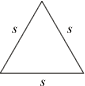index: click on a letter A B C D E F G H I J K L M N O P Q R S T U V W X Y Z A to Z index index: subject areas numbers & symbols sets, logic, proofs geometry algebra trigonometry advanced algebra & pre-calculus calculus advanced topics probability & statistics real world applications multimedia entrieswww.mathwords.com about mathwords website feedback

Equilateral Triangle

A triangle with three congruent sides.

Note: An equilateral triangle is also equiangular. In Euclidean geometry, the angles of an equilateral triangle each measure 60°.

 Equilateral Triangle s = length of a side